# Solving Equations with Solving Equations 10 3 Variables

• Slides: 27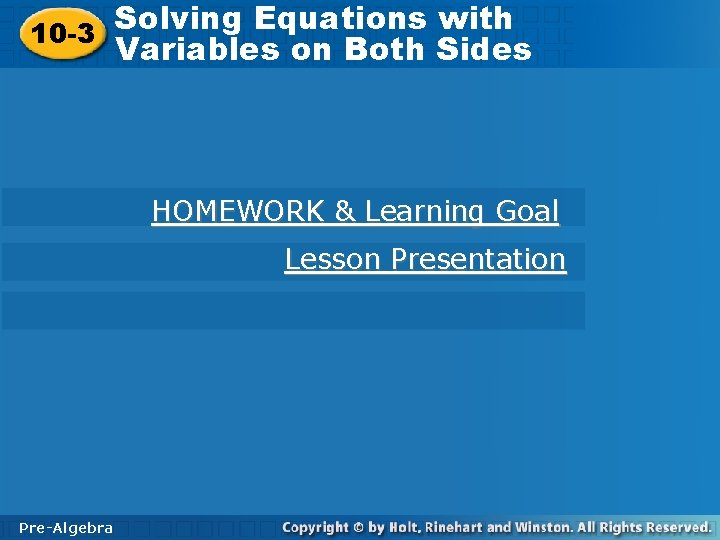Solving Equations with Solving Equations 10 -3 Variables on Both Sides HOMEWORK & Learning Goal Lesson Presentation Pre-Algebra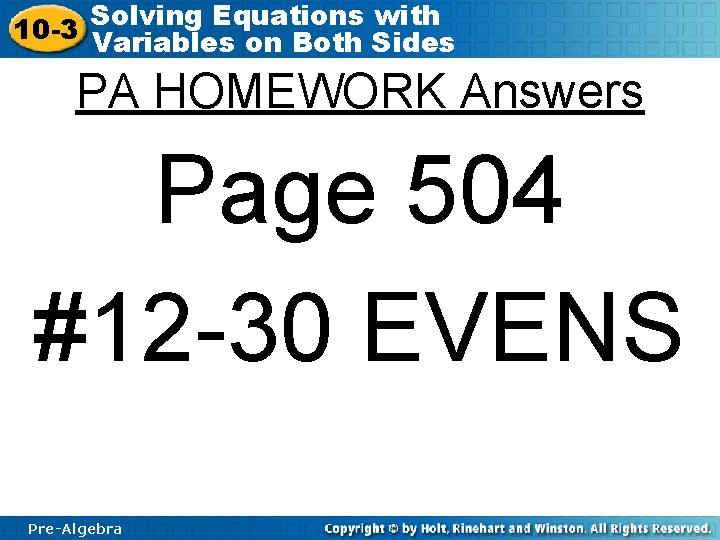Solving Equations with 10 -3 Variables on Both Sides PA HOMEWORK Answers Page 504 #12 -30 EVENS Pre-Algebra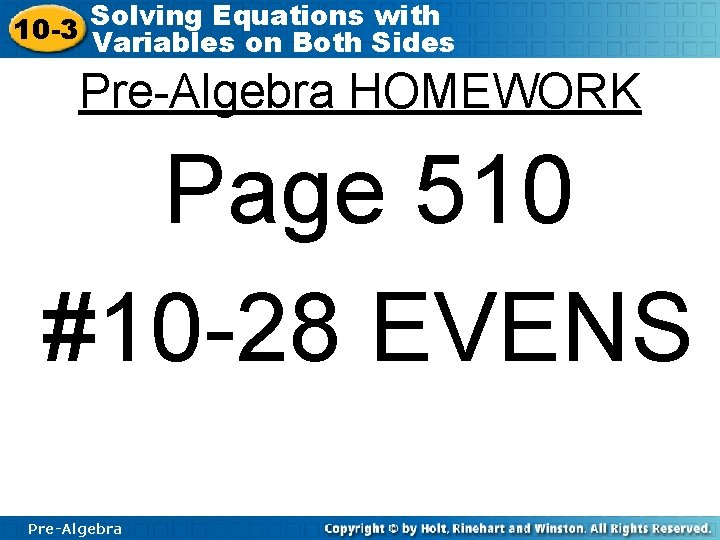Solving Equations with 10 -3 Variables on Both Sides Pre-Algebra HOMEWORK Page 510 #10 -28 EVENS Pre-AlgebraSolving Equations with 10 -3 Variables on Both Sides Our Learning Goal Students will be able to solve multi-step equations with multiple variables, solve inequalities and graph the solutions on a number line. Pre-AlgebraSolving Equations with 10 -3 Variables on Both Sides Our Learning Goal Assignments • Learn to solve two-step equations. • Learn to solve multistep equations. • Learn to solve equations with variables on both sides of the equal sign. • Learn to solve two-step inequalities and graph the solutions of an inequality on a number line. • Learn to solve an equation for a variable. • Learn to solve systems of equations. Pre-AlgebraSolving Equations with 10 -3 Variables on Both Sides Today’s Learning Goal Assignment Learn to solve equations with variables on both sides of the equal sign. Pre-AlgebraSolving Equations with 10 -3 Variables on Both Sides Solving Strategy for Variables! Some problems produce equations that have variables on both sides of the equal sign. Solving an equation with variables on both sides is similar to solving an equation with a variable on only one side. You can add or subtract a term containing a variable on both sides of an equation. Pre-AlgebraSolving Equations with 10 -3 Variables on Both Sides Additional Example 1 A: Solving Equations with Variables on Both Sides Solve. A. 4 x + 6 = x – 4 x 6 = – 3 x – 3 – 2 = x Pre-Algebra Subtract 4 x from both sides. Divide both sides by – 3.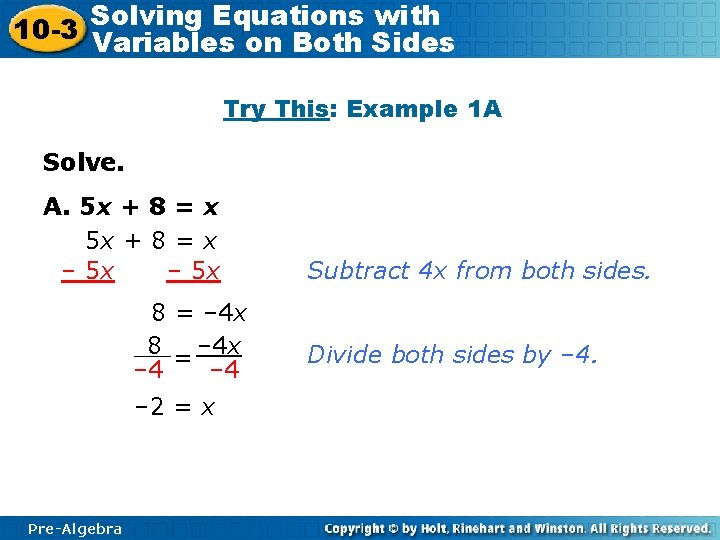Solving Equations with 10 -3 Variables on Both Sides Try This: Example 1 A Solve. A. 5 x + 8 = x – 5 x 8 = – 4 x – 4 – 2 = x Pre-Algebra Subtract 4 x from both sides. Divide both sides by – 4.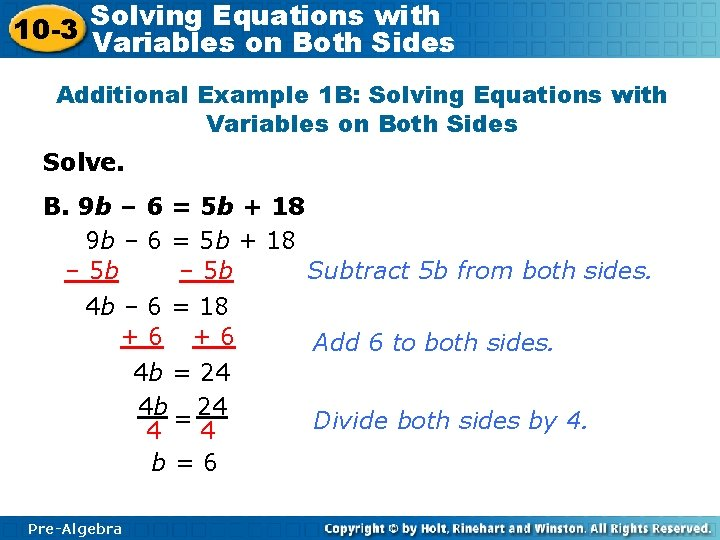Solving Equations with 10 -3 Variables on Both Sides Additional Example 1 B: Solving Equations with Variables on Both Sides Solve. B. 9 b – 6 = 5 b + 18 – 5 b Subtract 5 b from both sides. 4 b – 6 = 18 +6 +6 4 b = 24 4 4 b=6 Pre-Algebra Add 6 to both sides. Divide both sides by 4.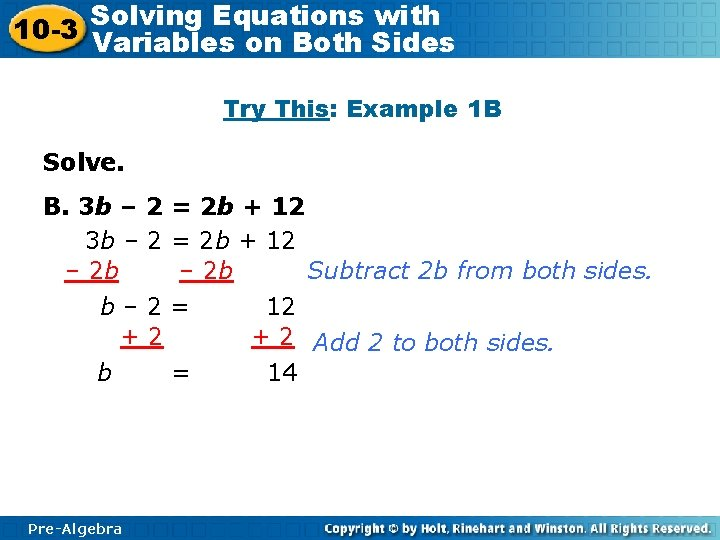Solving Equations with 10 -3 Variables on Both Sides Try This: Example 1 B Solve. B. 3 b – 2 = 2 b + 12 – 2 b Subtract 2 b from both sides. b– 2= +2 b = Pre-Algebra 12 + 2 Add 2 to both sides. 14Solving Equations with 10 -3 Variables on Both Sides Additional Example 1 C: Solving Equations with Variables on Both Sides Solve. C. 9 w + 3 – 9 w = 5 w + 7 + 4 w = 9 w + 7 Combine like terms. – 9 w Subtract 9 w from both sides. 3≠ 7 No solution. There is no number that can be substituted for the variable w to make the equation true. Pre-AlgebraSolving Equations with 10 -3 Variables on Both Sides Try This: Example 1 C Solve. C. 3 w + 1 – 3 w = 10 w + 8 – 7 w = 3 w + 8 Combine like terms. – 3 w Subtract 3 w from both sides. 1≠ 8 No solution. There is no number that can be substituted for the variable w to make the equation true. Pre-Algebra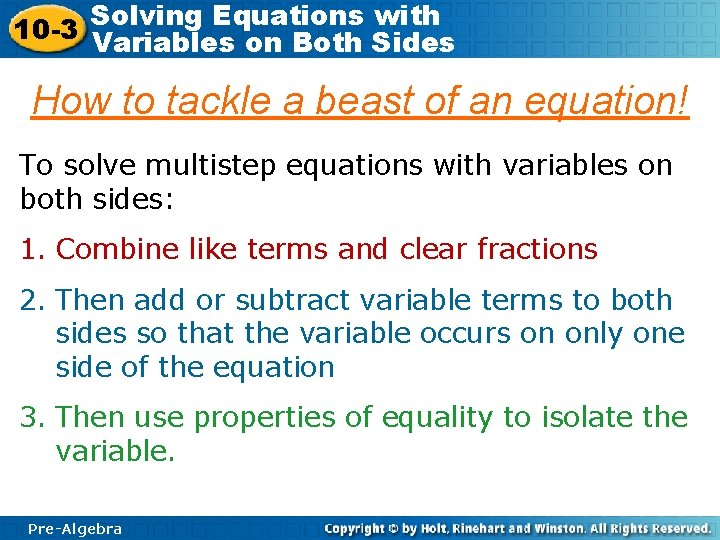Solving Equations with 10 -3 Variables on Both Sides How to tackle a beast of an equation! To solve multistep equations with variables on both sides: 1. Combine like terms and clear fractions 2. Then add or subtract variable terms to both sides so that the variable occurs on only one side of the equation 3. Then use properties of equality to isolate the variable. Pre-Algebra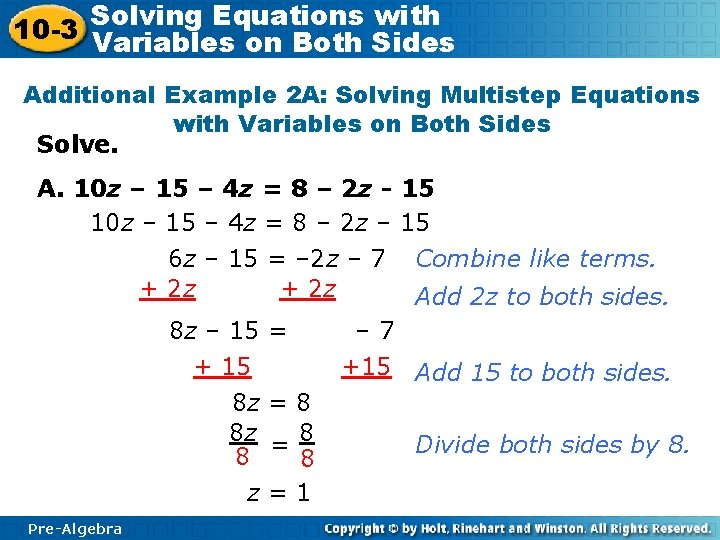Solving Equations with 10 -3 Variables on Both Sides Additional Example 2 A: Solving Multistep Equations with Variables on Both Sides Solve. A. 10 z – 15 – 4 z = 8 – 2 z – 6 z – 15 = – 2 z – 7 + 2 z 8 z – 15 + 15 8 z 8 z 8 z Pre-Algebra = =8 8 =1 15 15 Combine like terms. Add 2 z to both sides. – 7 +15 Add 15 to both sides. Divide both sides by 8.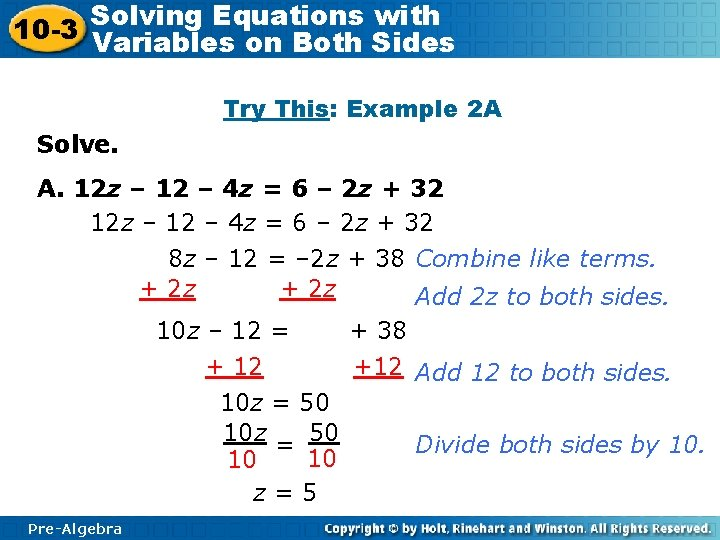Solving Equations with 10 -3 Variables on Both Sides Try This: Example 2 A Solve. A. 12 z – 12 – 4 z = 6 – 2 z + 32 8 z – 12 = – 2 z + 38 Combine like terms. + 2 z Add 2 z to both sides. 10 z – 12 = + 38 + 12 +12 Add 12 to both sides. 10 z = 50 10 z 50 Divide both sides by 10. = 10 10 z=5 Pre-Algebra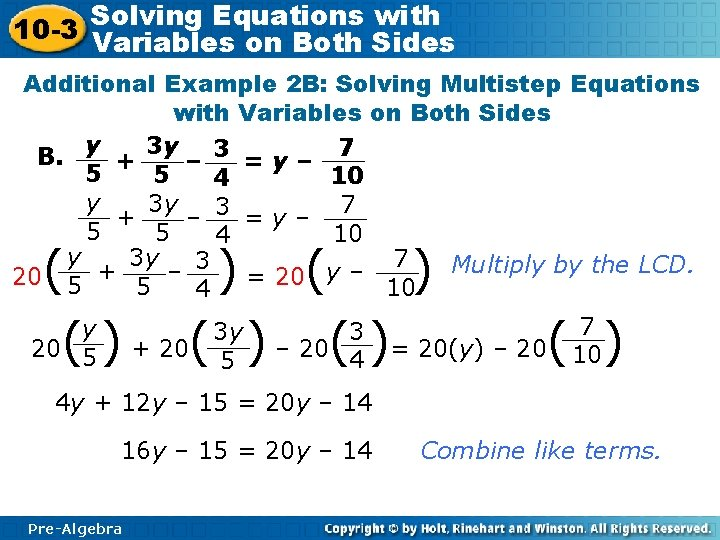Solving Equations with 10 -3 Variables on Both Sides Additional Example 2 B: Solving Multistep Equations with Variables on Both Sides B. y + 3 y – 3 = y – 7 5 5 10 4 y 3 y 7 + – 3 =y– 5 5 10 4 y 3 y 7 3 20 5 + 5 – = 20 y – 10 Multiply by the LCD. 4 ( ) 7 y 3 3 y 20(5 ) + 20( 5 ) – 20(4 )= 20(y) – 20( 10) 4 y + 12 y – 15 = 20 y – 14 16 y – 15 = 20 y – 14 Pre-Algebra Combine like terms.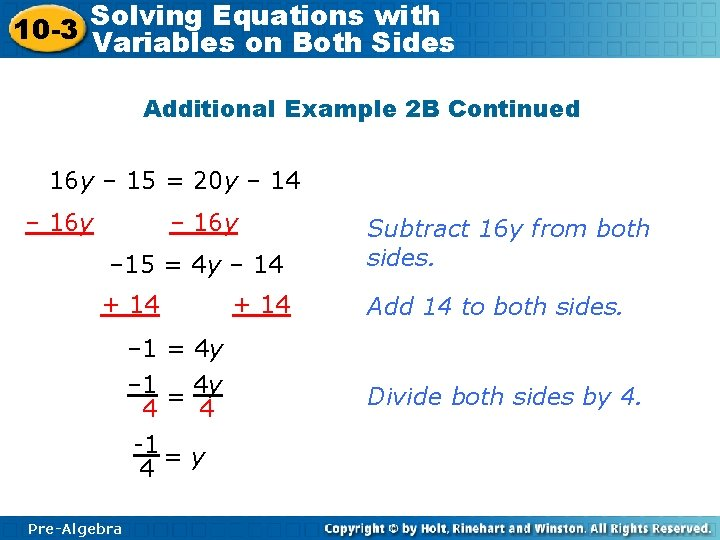Solving Equations with 10 -3 Variables on Both Sides Additional Example 2 B Continued 16 y – 15 = 20 y – 14 – 16 y – 15 = 4 y – 14 + 14 – 1 = 4 y 4 4 -1 = y 4 Pre-Algebra + 14 Subtract 16 y from both sides. Add 14 to both sides. Divide both sides by 4.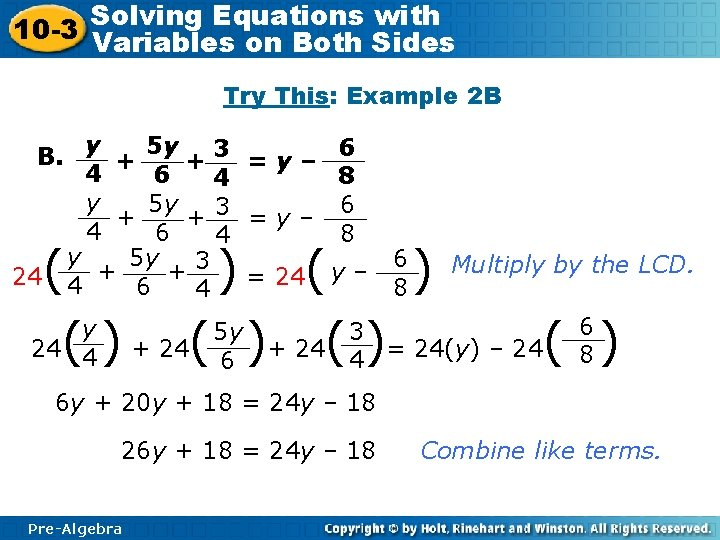Solving Equations with 10 -3 Variables on Both Sides Try This: Example 2 B B. y + 5 y + 3 = y – 4 6 4 y 5 y + +3 =y– 4 6 4 y 5 y 3 + + 24 4 = 24 6 8 6 8 ( ) ( y – ) Multiply by the LCD. 6 y 3 5 y 24(4 ) + 24( 6 )+ 24( 4)= 24(y) – 24( 8 ) 6 8 6 y + 20 y + 18 = 24 y – 18 26 y + 18 = 24 y – 18 Pre-Algebra Combine like terms.Solving Equations with 10 -3 Variables on Both Sides Try This: Example 2 B Continued 26 y + 18 = 24 y – 18 – 24 y 2 y + 18 = – 18 2 y = – 36 2 2 y = – 18 Pre-Algebra Subtract 24 y from both sides. Subtract 18 from both sides. Divide both sides by 2.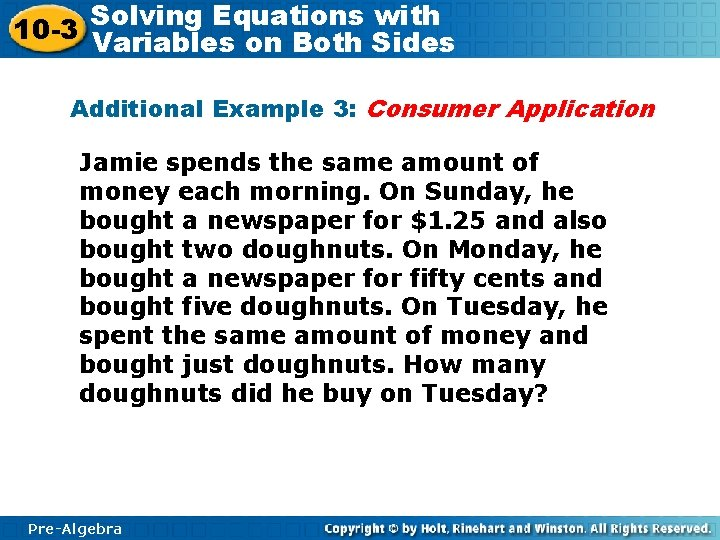Solving Equations with 10 -3 Variables on Both Sides Additional Example 3: Consumer Application Jamie spends the same amount of money each morning. On Sunday, he bought a newspaper for \$1. 25 and also bought two doughnuts. On Monday, he bought a newspaper for fifty cents and bought five doughnuts. On Tuesday, he spent the same amount of money and bought just doughnuts. How many doughnuts did he buy on Tuesday? Pre-Algebra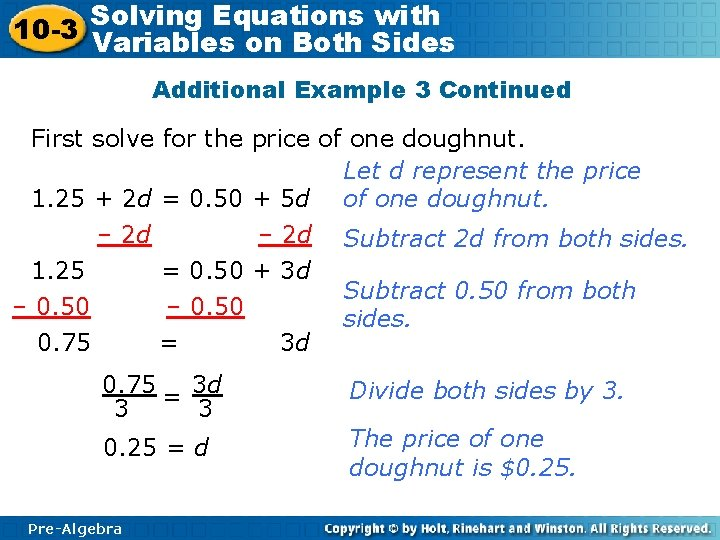Solving Equations with 10 -3 Variables on Both Sides Additional Example 3 Continued First solve for the price of one doughnut. Let d represent the price 1. 25 + 2 d = 0. 50 + 5 d of one doughnut. – 2 d Subtract 2 d from both sides. 1. 25 = 0. 50 + 3 d Subtract 0. 50 from both – 0. 50 sides. 0. 75 = 3 d 3 3 Divide both sides by 3. 0. 25 = d The price of one doughnut is \$0. 25. Pre-Algebra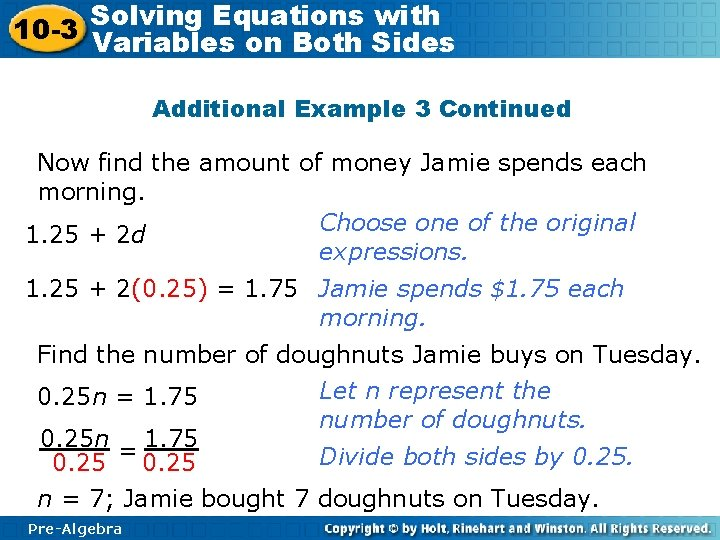Solving Equations with 10 -3 Variables on Both Sides Additional Example 3 Continued Now find the amount of money Jamie spends each morning. Choose one of the original 1. 25 + 2 d expressions. 1. 25 + 2(0. 25) = 1. 75 Jamie spends \$1. 75 each morning. Find the number of doughnuts Jamie buys on Tuesday. Let n represent the 0. 25 n = 1. 75 number of doughnuts. 0. 25 n = 1. 75 Divide both sides by 0. 25 n = 7; Jamie bought 7 doughnuts on Tuesday. Pre-AlgebraSolving Equations with 10 -3 Variables on Both Sides Try This: Example 3 Helene walks the same distance every day. On Tuesdays and Thursdays, she walks 2 laps on the track, and then walks 4 miles. On Mondays, Wednesdays, and Fridays, she walks 4 laps on the track and then walks 2 miles. On Saturdays, she just walks laps. How many laps does she walk on Saturdays? Pre-AlgebraSolving Equations with 10 -3 Variables on Both Sides Try This: Example 3 Continued First solve for distance around the track. Let x represent the distance 2 x + 4 = 4 x + 2 around the track. – 2 x Subtract 2 x from both sides. 4 = 2 x + 2 – 2 2 = 2 x 2 2 1=x Pre-Algebra Subtract 2 from both sides. Divide both sides by 2. The track is 1 mile around.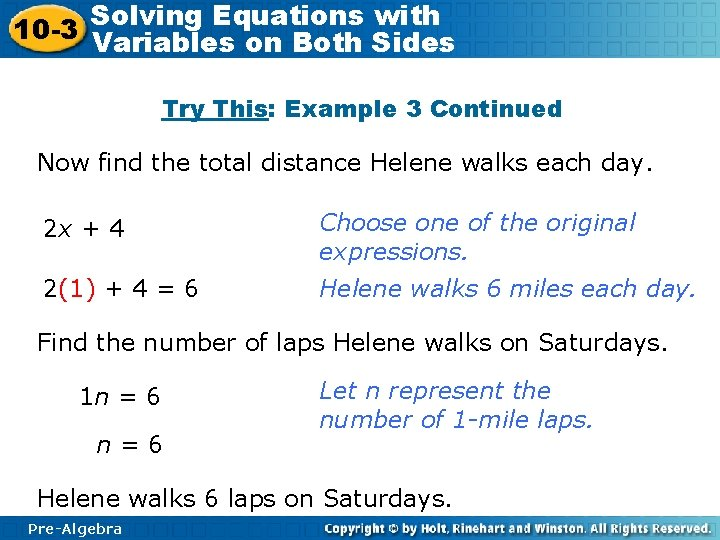Solving Equations with 10 -3 Variables on Both Sides Try This: Example 3 Continued Now find the total distance Helene walks each day. 2 x + 4 Choose one of the original expressions. 2(1) + 4 = 6 Helene walks 6 miles each day. Find the number of laps Helene walks on Saturdays. 1 n = 6 n=6 Let n represent the number of 1 -mile laps. Helene walks 6 laps on Saturdays. Pre-Algebra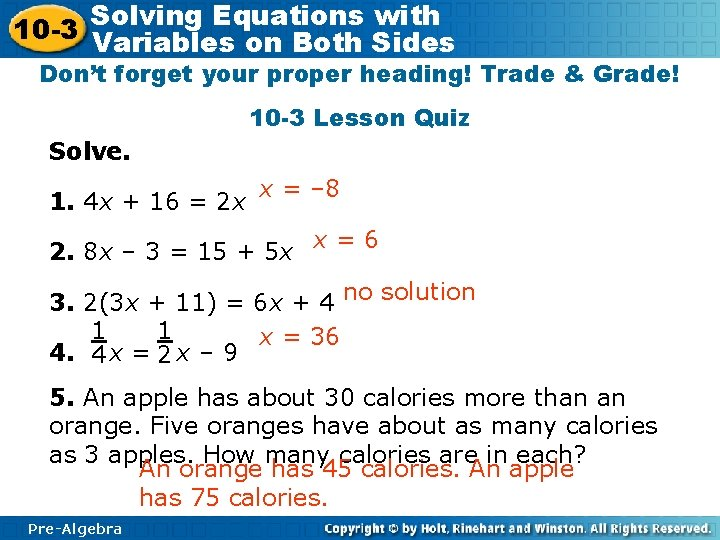Solving Equations with 10 -3 Variables on Both Sides Don’t forget your proper heading! Trade & Grade! 10 -3 Lesson Quiz Solve. 1. 4 x + 16 = 2 x x = – 8 2. 8 x – 3 = 15 + 5 x x=6 3. 2(3 x + 11) = 6 x + 4 no solution 1 1 x = 36 4. 4 x = 2 x – 9 5. An apple has about 30 calories more than an orange. Five oranges have about as many calories as 3 apples. How many calories are in each? An orange has 45 calories. An apple has 75 calories. Pre-Algebra